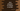# Java Program to reverse a number### Java program to reverse a number :

In this tutorial ,we will learn how to reverse a number in Java. First we will take the number as input from the user, then convert it to a string and reverse it . Then again we will convert it back to integer.

Following steps are using in the program :

1. Get the inger from the user using ‘Scanner’

2. Convert it to a string using ‘String.valueOf()’

3. Create an empty result string.

4. Scan the string converted number character by character using a ‘for’ loop from last to first

5. Append each character to the result string.

6. After the loop is completed, convert the result string to integer using ‘Integer.valueOf()’

7. Print out the result

### Java example Program :

``````import java.util.Scanner;

public class Main {

/**
* Utility functions
*/
static void println(String string) {
System.out.println(string);
}

static void print(String string) {
System.out.print(string);
}

public static void main(String[] args) {
Scanner sc = new Scanner(System.in);

println("Enter a number you want to rotate : ");
int number = sc.nextInt();

String strNumber = String.valueOf(number);

String reverseNumberString = "";

for (int i = strNumber.length() - 1; i >= 0; i--) {
reverseNumberString += strNumber.charAt(i);
}

int reverseNumber = Integer.valueOf(reverseNumberString);
println("Reverse of the number is " + reverseNumber);

}
}
``````

### Sample Output :

``````Enter a number you want to rotate :
125467
Reverse of the number is 764521

Enter a number you want to rotate :
562948
Reverse of the number is 849265

Enter a number you want to rotate :
1
Reverse of the number is 1

Enter a number you want to rotate :
54
Reverse of the number is 45
``````